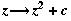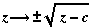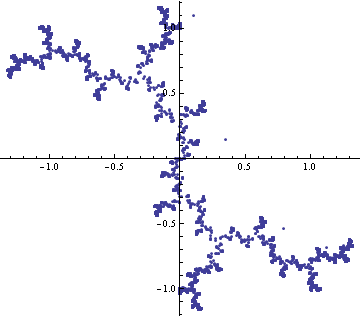# Inverse Julia Sets

Normally, a Julia set is calculated by iteratingfor a particular value of c (in this example, i). Alternatively we can iterate the inverse mappingrandomly picking either the positive or negative root.```
inverseJulia[z_] :=  Sqrt[z - I] /; Random[] < 0.5;

inverseJulia[z_] := -Sqrt[z - I];

complexPoints = NestList[inverseJulia, 0.1 + 1.1 I, 10000]

ListPlot[complexPoints /. Complex[a_, b_]->{a, b},
AspectRatio -> Automatic];```

Designed and rendered using Mathematica 3.0 for Apple Macintosh and (much later) 7.0 for Microsoft Windows.